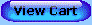For a lifetime of learning fun!

If you are unable to see the blue navigation buttons below, go to Contents for text links.1. Mathematical Ways of Thinking - The Path of the Billiard Ball, More Billiard-Ball Mathematics, Inductive Reasoning: Finding and Extending Patterns, The Limitations of Inductive Reasoning, Deductive Reasoning: Mathematical Proof, Number Tricks and Deductive Reasoning

2. Number Sequences - Arithmetic Sequences, Geometric Sequences, The Binary Sequence, The Sequence of Squares, The Sequence of Cubes, Fibonacci Sequence

3. Functions and Their Graphs - The Idea of a Function, Descartes and the Coordinate Graph, Functions with Line Graphs, Functions with Parabolic Graphs, More Functions with Curved Graphs, Interpolation and Extrapolation: Guessing Between and Beyond

4. Large Numbers and Logarithms - Large Numbers, Scientific Notation, An Introduction to Logarithms, Decimal Logarithms, Logarithms and Scientific Notation, Exponential Functions

5. Symmetry and Regular Figures - Symmetry, Regular Polygons, Mathematical Mosaics, Regular Polyhedra, Pyramids and Prisms

6. Mathematical Curves - The Circle and the Ellipse, The Parabola, The Hyperbola, The Sine Curve, Spirals, The Cycloid

7. Methods of Counting - The Fundamental Counting Principle, Permutations, More on Permutations, Combinations

8. The Mathematics of Chance - Probability: The Measure of Chance, Dice Games and Probability, Probabilities of Successive Events, Binomial Probability, Pascal's Triangle, Independent and Dependent Events, The Birthday Problem: Complimentary Events

9. An Introduction to Statistics - Organizing Data: Frequency Distributions, The Breaking of Ciphers and Codes: An Application of Statistics, Measures of Central Tendency, Measures of Variability, Displaying Data: Statistical Graphs, Collecting Data: Sampling

10. Topics in Topology - The Mathematics of Distortion, The Seven Bridges of Konigsberg: An Introduction to Networks, Euler Paths, Trees, The Moebius Strip and Other Surfaces

Appendix: - Basic Ideas and Operations, Angles and Their Measurement, The Distributive Rule, Signed Numbers, Percent